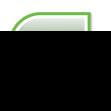0
Thanks

A few words of thanks would be greatly appreciated.

# Excel VBA - - Msg Box when cell meets criteria

## IssueHi this will probably be very simple for someone however i am having a nightmare day, all I want is a VBA formula which will bring up a message box when a cell is showing a specific criteria.

Can anyone help?

## Solution

This code assumes that what you are looking for is in column A.

```Private Sub Find_Criteria()

Dim i
Dim r
r = Range("A65536").End(xlUp).Row
i = 1

For i = i To r

If Range("A" & i) = "criteria" Then

MsgBox "Found" & " " & Range("A" & i).Address

End If

Next i

End Sub

If you are looking for certain criteria in a range of cells, then try something like this.

Private Sub Find_Criteria()

Dim i As Variant
Dim FindRange As Range
Set FindRange = Range("A1:K50")

For Each i In FindRange

If i = "criteria" Then

MsgBox "Found" & " " & i.Address

End If

Next i

End Sub```

## Note

Thanks to Helper for this tip on the forum.

0
Thanks

A few words of thanks would be greatly appreciated.